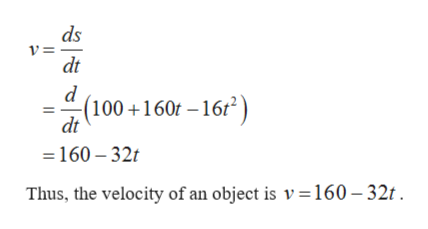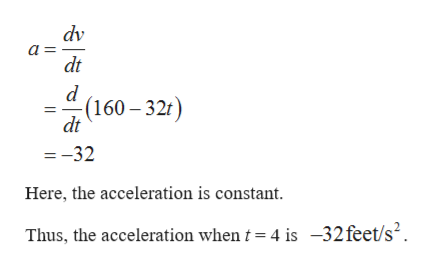# If the formula describing the distance s(in feet) an object travels as a function of time(in seconds) is s= 100+160t-16t^2what is the acceleration of the object when t=4?

Question
1 views

If the formula describing the distance s(in feet) an object travels as a function of time(in seconds) is

s= 100+160t-16t^2

what is the acceleration of the object when t=4?

check_circle

Step 1

The distance s(in feet) an object travels as a function is s = 100 + 160 t -16t2.

It is known that, the velocity of an object is calculated by the derivative of the distance with respect to t.help_outlineImage Transcriptioncloseds V= dt d -(100+160t -16/2 dt =160-32t Thus, the velocity of an object is v = 160 - 32t fullscreen
Step 2

Note that, the acceleration of an object is calculated by the ...help_outlineImage Transcriptionclosedv a dt -(160-32t) dt =-32 Here, the acceleration is constant Thus, the acceleration when t 4 is -32feet/s2. fullscreen

### Want to see the full answer?

See Solution

#### Want to see this answer and more?

Solutions are written by subject experts who are available 24/7. Questions are typically answered within 1 hour.*

See Solution
*Response times may vary by subject and question.
Tagged in

### Derivative